Calculus Volume 3

# Chapter 6

### Checkpoint

6.1

$12 i − j 12 i − j$

6.2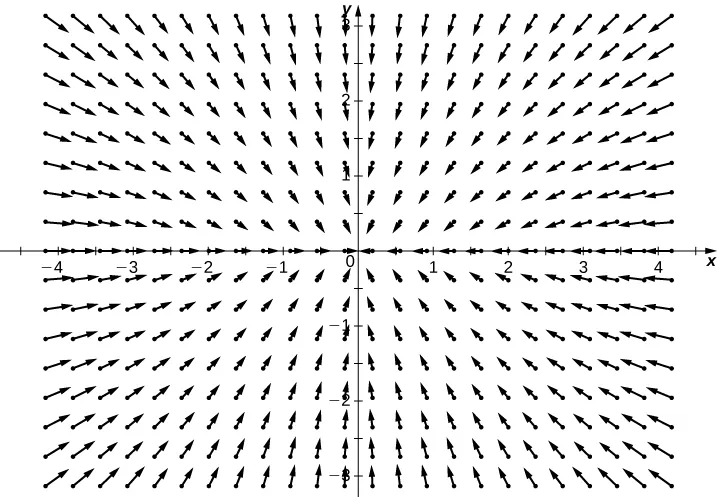6.3

Rotational6.4

$6565$ m/sec

6.5

No.

6.6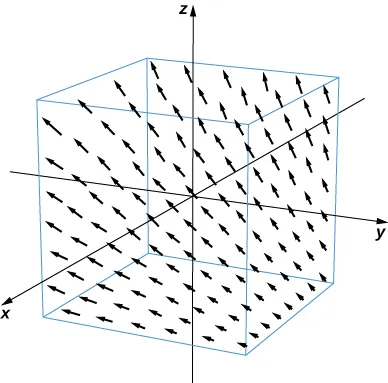6.7

$1.49063 × 10 −18 , 4.96876 × 10 −19 , 9.93752 × 10 −19 N 1.49063 × 10 −18 , 4.96876 × 10 −19 , 9.93752 × 10 −19 N$

6.8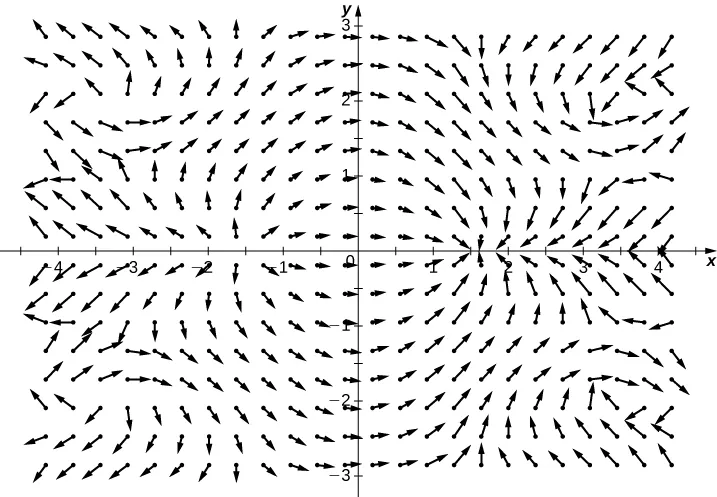6.9

No

6.10

$∇ f = v ∇ f = v$

6.11

$P y = x ≠ Q x = −2 x y P y = x ≠ Q x = −2 x y$

6.12

No

6.13

$2 2$

6.14

$2 10 π + 2 10 π 2 2 10 π + 2 10 π 2$

6.15

Both line integrals equal $−1000303.−1000303.$

6.16

$4 17 4 17$

6.17

$∫ C F · T d s ∫ C F · T d s$

6.18

$−26 −26$

6.19

0

6.20

$182π2182π2$ kg

6.21

3/2

6.22

$2 π 2 π$

6.23

0

6.24

Yes

6.25

The region in the figure is connected. The region in the figure is not simply connected.

6.26

2

6.27

If $C1C1$ and $C2C2$ represent the two curves, then $∫C1F.dr≠∫C2F.dr.∫C1F.dr≠∫C2F.dr.$

6.28

$f ( x , y ) = e x y 3 + x y f ( x , y ) = e x y 3 + x y$

6.29

$f ( x , y , z ) = 4 x 3 + sin y cos z + z f ( x , y , z ) = 4 x 3 + sin y cos z + z$

6.30

$f ( x , y , z ) = G x 2 + y 2 + z 2 f ( x , y , z ) = G x 2 + y 2 + z 2$

6.31

It is conservative.

6.32

$−10 π −10 π$

6.33

Negative

6.34

$45 2 45 2$

6.35

$4 3 4 3$

6.36

$3 π 2 3 π 2$

6.37

$g ( x , y ) = − x cos y g ( x , y ) = − x cos y$

6.38

No

6.39

$105 π 105 π$

6.40

$y − z 2 y − z 2$

6.41

Yes

6.42

All points on line $y=1.y=1.$

6.43

$− i − i$

6.44

$curl v = 0 curl v = 0$

6.45

No

6.46

Yes

6.47

Cylinder $x2+y2=4x2+y2=4$

6.48

Cone $x2+y2=z2x2+y2=z2$

6.49

$r(u,v)=〈ucosv,usinv,u〉,r(u,v)=〈ucosv,usinv,u〉,$ $0

6.50

Yes

6.51

$≈ 43.02 ≈ 43.02$

6.52

With the standard parameterization of a cylinder, Equation 6.18 shows that the surface area is $2πrh.2πrh.$

6.53

$2 π ( 2 + sinh −1 ( 1 ) ) 2 π ( 2 + sinh −1 ( 1 ) )$

6.54

24

6.55

0

6.56

$38.401 π ≈ 120.640 38.401 π ≈ 120.640$

6.57

$N ( x , y ) = 〈 − y 1 + x 2 + y 2 , − x 1 + x 2 + y 2 , 1 1 + x 2 + y 2 〉 N ( x , y ) = 〈 − y 1 + x 2 + y 2 , − x 1 + x 2 + y 2 , 1 1 + x 2 + y 2 〉$

6.58

0

6.59

400 kg/sec/m

6.60

$− 440 π 3 − 440 π 3$

6.61

Both integrals give $00$

6.62

$− π − π$

6.63

$3 2 3 2$

6.64

$curl E = 〈 x , y , −2 z 〉 curl E = 〈 x , y , −2 z 〉$

6.65

Both integrals equal $6π.6π.$

6.66

30

6.67

$9 ln ( 16 ) 9 ln ( 16 )$

6.68

$≈ 6.777 × 10 9 ≈ 6.777 × 10 9$

### Section 6.1 Exercises

1.

Vectors

3.

False

5.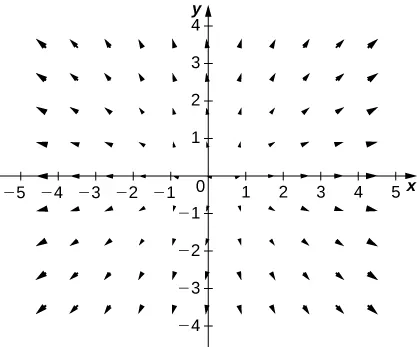7.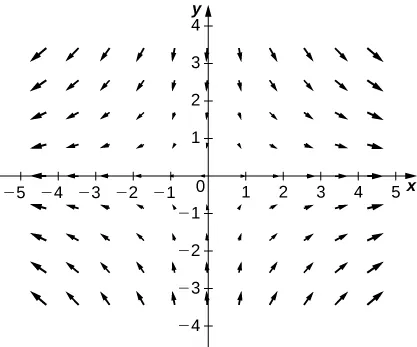9.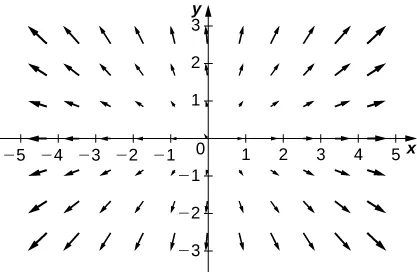11.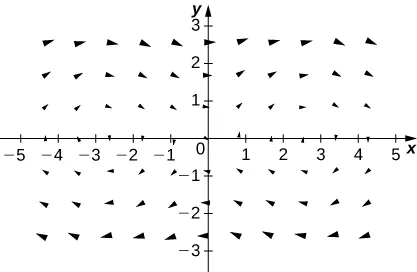13.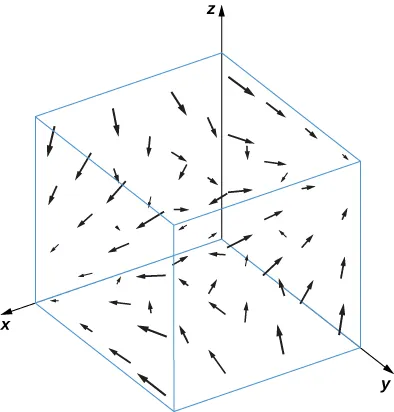15.

$F ( x , y ) = sin ( y ) i + ( x cos y − sin y ) j F ( x , y ) = sin ( y ) i + ( x cos y − sin y ) j$

17.

$F ( x , y , z ) = ( 2 x y + y ) i + ( x 2 + x + 2 y z ) j + y 2 k F ( x , y , z ) = ( 2 x y + y ) i + ( x 2 + x + 2 y z ) j + y 2 k$

19.

$F ( x , y ) = ( 2 x 1 + x 2 + 2 y 2 ) i + ( 4 y 1 + x 2 + 2 y 2 ) j F ( x , y ) = ( 2 x 1 + x 2 + 2 y 2 ) i + ( 4 y 1 + x 2 + 2 y 2 ) j$

21.

$F ( x , y ) = ( 1 − x ) i − y j ( 1 − x ) 2 + y 2 F ( x , y ) = ( 1 − x ) i − y j ( 1 − x ) 2 + y 2$

23.

$F ( x , y ) = - x i − y j x 2 + y 2 F ( x , y ) = - x i − y j x 2 + y 2$

25.

$F ( x , y ) = y i − x j F ( x , y ) = y i − x j$

27.

$F ( x , y ) = −10 ( x 2 + y 2 ) 3 / 2 ( x i + y j ) F ( x , y ) = −10 ( x 2 + y 2 ) 3 / 2 ( x i + y j )$

29.

$E = c | r | 2 r = c | r | r | r | E = c | r | 2 r = c | r | r | r |$

31.

$c ′ ( t ) = ( cos t , − sin t , e − t ) = F ( c ( t ) ) c ′ ( t ) = ( cos t , − sin t , e − t ) = F ( c ( t ) )$

33.

H

35.

d. $−F+G−F+G$

37.

a. $F+GF+G$

### Section 6.2 Exercises

39.

True

41.

False

43.

False

45.

$∫ C ( x − y ) d s = 10 ∫ C ( x − y ) d s = 10$

47.

$∫ C x y 4 d s = 8192 5 ∫ C x y 4 d s = 8192 5$

49.

$W = 8 W = 8$

51.

$W = 3 π 4 W = 3 π 4$

53.

$W = π W = π$

55.

$∫ C F · d r = 4 ∫ C F · d r = 4$

57.

$∫ C y z d x + x z d y + x y d z = −1 ∫ C y z d x + x z d y + x y d z = −1$

59.

$∫ C ( y 2 ) d x + ( x ) d y = 245 6 ∫ C ( y 2 ) d x + ( x ) d y = 245 6$

61.

$∫ C x y d x + y d y = 190 3 ∫ C x y d x + y d y = 190 3$

63.

$∫ C y 2 x 2 − y 2 d s = 2 ln 5 ∫ C y 2 x 2 − y 2 d s = 2 ln 5$

65.

$W = −66 W = −66$

67.

$W = −10 π 2 W = −10 π 2$

69.

$W = 2 W = 2$

71.

a. $W=11;W=11;$ b. $W=394;W=394;$ c. No

73.

$W = 2 π W = 2 π$

75.

$∫ C F · d s = 25 5 + 1 120 ∫ C F · d s = 25 5 + 1 120$

77.

$∫ C y 2 d x + ( x y − x 2 ) d y = 6.15 ∫ C y 2 d x + ( x y − x 2 ) d y = 6.15$

79.

$∫ γ x e y d s ≈ 7.157 ∫ γ x e y d s ≈ 7.157$

81.

$∫ γ ( y 2 − x y ) d x ≈ −1.379 ∫ γ ( y 2 − x y ) d x ≈ −1.379$

83.

$∫ C F · d r ≈ −1.133 ∫ C F · d r ≈ −1.133$

85.

$∫ C F · d r ≈ 2 2 . 8 5 7 ∫ C F · d r ≈ 2 2 . 8 5 7$

87.

$flux = − 1 3 flux = − 1 3$

89.

$flux = −20 flux = −20$

91.

$flux = 0 flux = 0$

93.

$m = 4 π ρ 5 m = 4 π ρ 5$

95.

$W = 0 W = 0$

97.

$W = k 2 W = k 2$

### Section 6.3 Exercises

99.

True

101.

True

103.

$∫ C F · d r = 24 ∫ C F · d r = 24$

105.

$∫ C F · d r = e − 3 π 2 ∫ C F · d r = e − 3 π 2$

107.

Not conservative

109.

Conservative, $f(x,y)=3x2+5xy+2y2f(x,y)=3x2+5xy+2y2$

111.

Conservative, $f(x,y)=yex+xsin(y)f(x,y)=yex+xsin(y)$

113.

$∫ C ( 2 y d x + 2 x d y ) = 32 ∫ C ( 2 y d x + 2 x d y ) = 32$

115.

$F ( x , y ) = ( 10 x + 3 y ) i + ( 3 x + 20 y ) j F ( x , y ) = ( 10 x + 3 y ) i + ( 3 x + 20 y ) j$

117.

F is not conservative.

119.

F is conservative and a potential function is $f(x,y,z)=xyez.f(x,y,z)=xyez.$

121.

F is conservative and a potential function is $f(x,y,z)=z2–z–xy.f(x,y,z)=z2–z–xy.$

123.

F is conservative and a potential function is $f(x,y,z)=x2y+y2z.f(x,y,z)=x2y+y2z.$

125.

F is conservative and a potential function is $f(x,y)=ex2yf(x,y)=ex2y$

127.

$∫ C F · d r = e 2 + 2 ∫ C F · d r = e 2 + 2$

129.

$∫ C F · d r = -2 ∫ C F · d r = -2$

131.

$∮ C 1 G · d r = −8 π ∮ C 1 G · d r = −8 π$

133.

$∮ C 2 F · d r = 7 ∮ C 2 F · d r = 7$

135.

$∫ C F · d r = 159 ∫ C F · d r = 159$

137.

$∫ C F · d r = −1 ∫ C F · d r = −1$

139.

$4 × 10 31 erg 4 × 10 31 erg$

141.

$∫ C F · d s = 0.4687 ∫ C F · d s = 0.4687$

143.

$circulation = π a 2 and flux = 0 circulation = π a 2 and flux = 0$

### Section 6.4 Exercises

147.

$∫ C 2 x y d x + ( x + y ) d y = 32 3 ∫ C 2 x y d x + ( x + y ) d y = 32 3$

149.

$∫ C sin x cos y d x + ( x y + cos x sin y ) d y = 1 12 ∫ C sin x cos y d x + ( x y + cos x sin y ) d y = 1 12$

151.

$∮ C ( − y d x + x d y ) = π ∮ C ( − y d x + x d y ) = π$

153.

$∫ C x e −2 x d x + ( x 4 + 2 x 2 y 2 ) d y = 0 ∫ C x e −2 x d x + ( x 4 + 2 x 2 y 2 ) d y = 0$

155.

$∮Cy3dx−x3ydy=−20π∮Cy3dx−x3ydy=−20π$

157.

$∮ C − x 2 y d x + x y 2 d y = 8 π ∮ C − x 2 y d x + x y 2 d y = 8 π$

159.

$∮ C ( x 2 + y 2 ) d x + 2 x y d y = 0 ∮ C ( x 2 + y 2 ) d x + 2 x y d y = 0$

161.

$A = 19 π A = 19 π$

163.

$A = 3 8 π A = 3 8 π$

165.

$∫ C + ( y 2 + x 3 ) d x + x 4 d y = 0 ∫ C + ( y 2 + x 3 ) d x + x 4 d y = 0$

167.

$A = 9 π 8 A = 9 π 8$

169.

$A = 8 3 5 A = 8 3 5$

171.

$∫ C ( x 2 y − 2 x y + y 2 ) d s = - 5 6 ∫ C ( x 2 y − 2 x y + y 2 ) d s = - 5 6$

173.

$∫ C x d x + y d y x 2 + y 2 = 2 π ∫ C x d x + y d y x 2 + y 2 = 2 π$

175.

$W = 225 2 W = 225 2$

177.

$W = 12 π W = 12 π$

179.

$W = 2 π W = 2 π$

181.

$∮ C y 2 d x + x 2 d y = 1 3 ∮ C y 2 d x + x 2 d y = 1 3$

183.

$∫ C 1 + x 3 d x + 2 x y d y = -3 ∫ C 1 + x 3 d x + 2 x y d y = -3$

185.

$∫ C ( 3 y − e sin x ) d x + ( 7 x + y 4 + 1 ) d y = 36 π ∫ C ( 3 y − e sin x ) d x + ( 7 x + y 4 + 1 ) d y = 36 π$

187.

$∮ C F · d r = 2 ∮ C F · d r = 2$

189.

$∮ C ( y + x ) d x + ( x + sin y ) d y = 0 ∮ C ( y + x ) d x + ( x + sin y ) d y = 0$

191.

$∮ C x y d x + x 3 y 3 d y = 22 21 ∮ C x y d x + x 3 y 3 d y = 22 21$

193.

$∮ C F · d r = 15 π 4 ∮ C F · d r = 15 π 4$

195.

$∫ C sin ( x + y ) d x + cos ( x + y ) d y = 4 ∫ C sin ( x + y ) d x + cos ( x + y ) d y = 4$

197.

$∫ C F · d r = π ∫ C F · d r = π$

199.

$∮ C F · n ^ d s = 4 ∮ C F · n ^ d s = 4$

201.

$∮ C F · n d s = 0 ∮ C F · n d s = 0$

203.

$∫ C [ − y 3 + sin ( x y ) + x y cos ( x y ) ] d x + [ x 3 + x 2 cos ( x y ) ] d y = 4.7124 ∫ C [ − y 3 + sin ( x y ) + x y cos ( x y ) ] d x + [ x 3 + x 2 cos ( x y ) ] d y = 4.7124$

205.

$∮ C ( y + e x ) d x + ( 2 x + cos ( y 2 ) ) d y = 1 3 ∮ C ( y + e x ) d x + ( 2 x + cos ( y 2 ) ) d y = 1 3$

### Section 6.5 Exercises

207.

False

209.

True

211.

True

213.

$curl F = i + x 2 j + y 2 k curl F = i + x 2 j + y 2 k$

215.

$curl F = ( x z 2 − x y 2 ) i + ( x 2 y − y z 2 ) j + ( y 2 z − x 2 z ) k curl F = ( x z 2 − x y 2 ) i + ( x 2 y − y z 2 ) j + ( y 2 z − x 2 z ) k$

217.

$curl F = i + j + k curl F = i + j + k$

219.

$curl F = − y i − z j − x k curl F = − y i − z j − x k$

221.

$curl F = 0 curl F = 0$

223.

$div F = 3 y z 2 + 2 y sin z + 2 x e 2 z div F = 3 y z 2 + 2 y sin z + 2 x e 2 z$

225.

$div F = 2 ( x + y + z ) div F = 2 ( x + y + z )$

227.

$div F = 1 x 2 + y 2 div F = 1 x 2 + y 2$

229.

$div F = a + b div F = a + b$

231.

$div F = x + y + z div F = x + y + z$

233.

Harmonic

235.

$div ( F × G ) = 2 z + 3 x div ( F × G ) = 2 z + 3 x$

237.

$div F = 2 r 2 div F = 2 r 2$

239.

$curl r = 0 curl r = 0$

241.

$curl r r 3 = 0 curl r r 3 = 0$

243.

$curl F = 2 x x 2 + y 2 k curl F = 2 x x 2 + y 2 k$

245.

$div F = 0 div F = 0$

247.

$div F = 2 − 2 e −6 div F = 2 − 2 e −6$

249.

$div F = 0 div F = 0$

251.

$curl F = j − 3 k curl F = j − 3 k$

253.

$curl F = 2 j − k curl F = 2 j − k$

255.

$a = 3 a = 3$

257.

F is conservative.

259.

$div F = cosh x + sinh y − x y div F = cosh x + sinh y − x y$

261.

$( b z − c y ) i ( c x − a z ) j + ( a y − b x ) k ( b z − c y ) i ( c x − a z ) j + ( a y − b x ) k$

263.

$curl F = 2 ω curl F = 2 ω$

265.

$F×GF×G$ does not have zero divergence.

267.

$∇ · F = −200 k [ 1 + 2 ( x 2 + y 2 + z 2 ) ] e − x 2 + y 2 + z 2 ∇ · F = −200 k [ 1 + 2 ( x 2 + y 2 + z 2 ) ] e − x 2 + y 2 + z 2$

### Section 6.6 Exercises

269.

True

271.

True

273.

$r(u,v)=〈u,v,2−3u+2v〉r(u,v)=〈u,v,2−3u+2v〉$ for $−∞≤u<∞−∞≤u<∞$ and $−∞≤v<∞.−∞≤v<∞.$

275.

$r(u,v)=〈u,v,13(16−2u+4v)〉r(u,v)=〈u,v,13(16−2u+4v)〉$ for $|u|<∞|u|<∞$ and $|v|<∞.|v|<∞.$

277.

$r(u,v)=〈3cosu,3sinu,v〉r(u,v)=〈3cosu,3sinu,v〉$ for $0≤u≤π2,0≤v≤30≤u≤π2,0≤v≤3$

279.

$A = 87.9646 A = 87.9646$

281.

$∬ S z d S = 8 π ∬ S z d S = 8 π$

283.

$∬ S ( x 2 + y 2 ) z d S = 16 π ∬ S ( x 2 + y 2 ) z d S = 16 π$

285.

$∬ S F · N d S = 4 π 3 ∬ S F · N d S = 4 π 3$

287.

$m ≈ 13.0639 m ≈ 13.0639$

289.

$m ≈ 228.5313 m ≈ 228.5313$

291.

$∬ S g d S = 3 14 ∬ S g d S = 3 14$

293.

$∬ S ( x 2 + y − z ) d S ≈ 0.9617 ∬ S ( x 2 + y − z ) d S ≈ 0.9617$

295.

$∬ S ( x 2 + y 2 ) d S = 4 π 3 ∬ S ( x 2 + y 2 ) d S = 4 π 3$

297.

$∬ S x 2 z d S = 1023 2 π 5 ∬ S x 2 z d S = 1023 2 π 5$

299.

$∬ S ( z + y ) d S ≈ 10.1 ∬ S ( z + y ) d S ≈ 10.1$

301.

$m = π a 3 m = π a 3$

303.

$∬ S F · N d S = 13 24 ∬ S F · N d S = 13 24$

305.

$∬ S F · N d S = 3 4 ∬ S F · N d S = 3 4$

307.

$∫ 0 8 ∫ 0 6 ( 4 − 3 y + 1 16 y 2 + z ) ( 1 4 17 ) d z d y ∫ 0 8 ∫ 0 6 ( 4 − 3 y + 1 16 y 2 + z ) ( 1 4 17 ) d z d y$

309.

$∫ 0 2 ∫ 0 6 [ x 2 − 2 ( 8 − 4 x ) + z ] 17 d z d x ∫ 0 2 ∫ 0 6 [ x 2 − 2 ( 8 − 4 x ) + z ] 17 d z d x$

311.

$∬ S ( x 2 z + y 2 z ) d S = π a 5 2 ∬ S ( x 2 z + y 2 z ) d S = π a 5 2$

313.

$∬ S x 2 y z d S = 171 14 ∬ S x 2 y z d S = 171 14$

315.

$∬ S y z d S = 2 π 4 ∬ S y z d S = 2 π 4$

317.

$∬ S ( x i + y j ) · d S = 16 π ∬ S ( x i + y j ) · d S = 16 π$

319.

$m = π a 7 192 m = π a 7 192$

321.

$F ≈ 4.57 lb . F ≈ 4.57 lb .$

323.

$8 π a 8 π a$

325.

The net flux is zero.

### Section 6.7 Exercises

327.

$∬ S ( curl F · N ) d S = π a 2 ∬ S ( curl F · N ) d S = π a 2$

329.

$∬ S ( curl F · N ) d S = 18 π ∬ S ( curl F · N ) d S = 18 π$

331.

$∬ S ( curl F · N ) d S = −8 π ∬ S ( curl F · N ) d S = −8 π$

333.

$∬ S ( curl F · N ) d S = 0 ∬ S ( curl F · N ) d S = 0$

335.

$∫ C F · d S = 0 ∫ C F · d S = 0$

337.

$∫ C F · d S = −9.4248 ∫ C F · d S = −9.4248$

339.

$∬ S curl F · d S = 0 ∬ S curl F · d S = 0$

341.

$∬ S curl F · d S = 2.6667 ∬ S curl F · d S = 2.6667$

343.

$∬ S ( curl F · N ) d S = − 1 6 ∬ S ( curl F · N ) d S = − 1 6$

345.

$∫ C ( 1 2 y 2 d x + z d y + x d z ) = − π 4 ∫ C ( 1 2 y 2 d x + z d y + x d z ) = − π 4$

347.

$∬ S ( curl F · N ) d S = 3 π ∬ S ( curl F · N ) d S = 3 π$

349.

$∫ C ( c k × R ) · d S = 2 π c ∫ C ( c k × R ) · d S = 2 π c$

351.

$∬ S curl F · d S = 0 ∬ S curl F · d S = 0$

353.

$∫ C F · d r = −4 ∫ C F · d r = −4$

355.

$∬ S curl F · d S = 0 ∬ S curl F · d S = 0$

357.

$∬ S curl F · d S = −36 π ∬ S curl F · d S = −36 π$

359.

$∬ S curl F · N = 0 ∬ S curl F · N = 0$

361.

$∫ C F · d r = 0 ∫ C F · d r = 0$

363.

$∬ S curl ( F ) · d S = 84.8230 ∬ S curl ( F ) · d S = 84.8230$

365.

$A = ∬ S ( ∇ × F ) · n d S = 0 A = ∬ S ( ∇ × F ) · n d S = 0$

367.

$∬ S ( ∇ × F ) · n d S = 2 π ∬ S ( ∇ × F ) · n d S = 2 π$

369.

$C = π ( cos φ − sin φ ) C = π ( cos φ − sin φ )$

371.

$∮ C F · d r = 48 π ∮ C F · d r = 48 π$

373.

$∬ S ( ∇ × F ) · n = 0 ∬ S ( ∇ × F ) · n = 0$

375.

0

### Section 6.8 Exercises

377.

$∫ S F · n d s = 75.3982 ∫ S F · n d s = 75.3982$

379.

$∫ S F · n d s = 127.2345 ∫ S F · n d s = 127.2345$

381.

$∫ S F · n d s = 37.6991 ∫ S F · n d s = 37.6991$

383.

$∫ S F · n d s = 9 π a 4 2 ∫ S F · n d s = 9 π a 4 2$

385.

$∬ S F · d S = π 3 ∬ S F · d S = π 3$

387.

$∬ S F · d S = 0 ∬ S F · d S = 0$

389.

$∬ S F · d S = 241.2743 ∬ S F · d S = 241.2743$

391.

$∬ D F · d S = − π ∬ D F · d S = − π$

393.

$∬ S F · d S = 2 π 3 ∬ S F · d S = 2 π 3$

395.

$16 6 π 16 6 π$

397.

$− 128 3 π − 128 3 π$

399.

$−703.7168 −703.7168$

401.

20

403.

$∬ S F · d S = 8 ∬ S F · d S = 8$

405.

$∬ S F · N d S = 1 8 ∬ S F · N d S = 1 8$

407.

$∬ S ‖ R ‖ R · n d S = 4 π a 4 ∬ S ‖ R ‖ R · n d S = 4 π a 4$

409.

$∭ R z 2 d V = 4 π 15 ∭ R z 2 d V = 4 π 15$

411.

$∬ S F · d S = 6.5759 ∬ S F · d S = 6.5759$

413.

$∬ S F · d S = 21 ∬ S F · d S = 21$

415.

$∬ S F · d S = 72 ∬ S F · d S = 72$

417.

$∬ S F · d S = −33.5103 ∬ S F · d S = −33.5103$

419.

$∬ S F · d S = π a 4 b 2 ∬ S F · d S = π a 4 b 2$

421.

$∬ S F · d S = 5 2 π ∬ S F · d S = 5 2 π$

423.

$∬ S F · d S = 531 π 32 ∬ S F · d S = 531 π 32$

425.

$− ( 1 − e −1 ) − ( 1 − e −1 )$

### Review Exercises

427.

False

429.

False

431.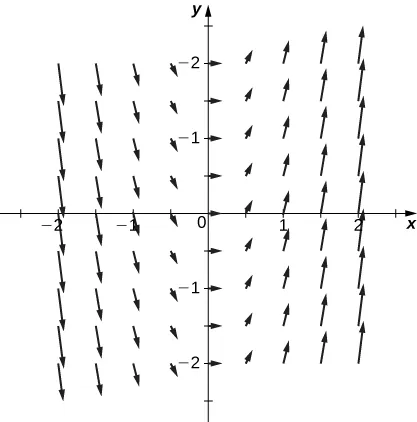433.

Conservative, $f(x,y)=xy−2eyf(x,y)=xy−2ey$

435.

Conservative, $f(x,y,z)=x2y+y2z+z2xf(x,y,z)=x2y+y2z+z2x$

437.

$− 16 3 − 16 3$

439.

$32 2 9 ( 3 3 − 1 ) 32 2 9 ( 3 3 − 1 )$

441.

Divergence: $ex+xexy+xyexyz,ex+xexy+xyexyz,$ curl: $xzexyzi−yzexyzj+yexykxzexyzi−yzexyzj+yexyk$

443.

$−18 π −18 π$

445.

$− π − π$

447.

$24 π 24 π$

449.

$2 ( 2 2 + π ) = 4 + π 2 2 ( 2 2 + π ) = 4 + π 2$

451.

$8 π / 3 8 π / 3$

Order a print copy

As an Amazon Associate we earn from qualifying purchases.